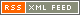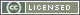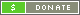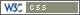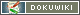# Allan Variance

From the Time-Nuts mailing list:

Well, I have been sort of staying out of the recent discussions regarding oscillator stability measurements, ADEV, etc., but here goes my two cents worth. I hope it doesn't muddy the waters too much and is somewhat helpful.

There is a fundamental problem with trying to do ADEV (Allan Deviation) with a counter that has a gated measurement engine (all standard counters). The problem is that when the count engine gate closes (so the counter can compute the number of events, report the result as a frequency, and clear its registers), the counter is blind (dead time) to any signal at the input.

Allan deviation REQUIRES than a minimum number of measurements be made back-to-back (no dead time between measurements) in order to capture nondeterministic fluctuations of the signal you're trying to measure. This requires a measuring device (counter) that continuously records ALL events. It does this by time-stamping a fixed period in a separate register in the counter along with the input register (the signal being measured), and a time-base register that records the frequency the signal is to be compared with. There is no ‘gate’ as such in a time-stamping counter.

Most stability measurements, longer than say 100 seconds, are made in the frequency domain because energy changes, as a result of heat, are by far the predominate cause of stability (aging) issues (see below). Stability issues within shorter time periods, say <100 sec., are often nondeterministic, and cannot be accurately quantified in the frequency domain, but must be measured in the time domain, using statistical weighting. In other words, unpredictable, and often little understood, events (Shot and thermal noise in the active devices, random variations in the frequency-determining elements, cosmic rays, etc.) often predominate short term stability measurements and must be described statistically, as their occurrence and duration are random. Allan deviation is a widely accepted time-domain statistical measurement whose calculated results compare well with the more common frequency domain measurement of longer time periods.

Allan deviation measurements are based on the sample variance of the fractional-frequency fluctuations. Without specifying the number of samples N, and the repetition interval T, for measurements of duration t, the measure of frequency stability is dimensionless and would converge to a meaningless limit. Secondly, some actual noise processes contain substantial fractions of the total noise power in the instantaneous fractional-frequency range below one cycle per year. In order to improve compatibility of data, it is important to specify a particular N and T. The Allan variance chooses N=2 and T=t (i.e. no dead time between measurements). A good estimate can be obtained by a limited number, m, of measurements (m=*100). Root Allen variance is expressed as a quantity divided by the square of the measurements of duration t, (i.e., 3 x 10-11/*t).

Classical variance diverges for commonly observed noise processes, such as random walk (i.e., the variance increases with an increasing number of data points). The advantage with the Allan variance is that it:

• converges for all noise processes observed in precision oscillators;
• has straightforward relationship to power law spectral density (spectral density of the frequency fluctuations);
• is easy to compute, and;
• is faster and more accurate in estimating noise processes than the Fast Fourier Transform.

Aging in quartz crystal oscillators is caused by changes in either the quartz crystal itself or the associated components found in the oscillator assembly. Aging is the result of a combination of several factors having complex, and only partially understood, components that effect the aging specification. Effects can include the cut (orientation) of the crystal; vibration modes; frequency of cut size; temperature of operation and variations of temperature; drive energy; gravity; physical orientation; shock; electromagnetic interference; diffusion of impurities and the outgassing of the quartz crystal; the glass or ceramic base; the adhesive used to mount the quartz; metal migration from the electrodes into the quartz surface; stress relief of the crystal mounts; changes of electric component values over time; and, voltage regulation.

This is probably more information than you really wanted, but oh well, enjoy.

Tom Duckworth

`Folks,` `I asked about 3 cornered comparisons some time ago, and now have plenty of data to start exploring, and way more than the 3 oscillators I had when I asked six months ago.`

`Apart from Stable32, which I do not have access to, are there any free tools which will allow me to perform 3 cornered comparisons between my oscillators?`

`Thanks,`

`david`

I use Excel on the { tau, ADEV } pairs when I want to get fancy, or just a calculator for something quick. You can sometimes simply eyeball it on a composite log-log adev plot.

I'll give the formula is below, but to understand, first consider this backwards example:

Suppose for some tau the ADEV of three oscillators is 6e-12, 8e-12, and 10e-12, respectively. But – you don't know that yet – because all you have is pairwise measurements. The assumption is that noise is rms additive. Let's do the numbers:

When you measure A vs. B you should get 1.00e-11, since that is sqrt( 6e-12 ^ 2 + 8e-12 ^ 2 ).

When you measure B vs. C you should get 1.28e-11, since that is sqrt( 8e-12 ^ 2 + 10e-12 ^ 2 ).

When you measure C vs. A you should get 1.17e-11, since that is sqrt( 10e-12 ^ 2 + 6e-12 ^ 2 ).

So given your three ADEV measurement pairs (AB=1.00e-11, BC=1.28e-11, AC=1.17e-11) you just work backwards to compute ADEV for A, B, and C, as in:

A = 0.707 * sqrt(+ AB*AB - BC*BC + AC*AC)
B = 0.707 * sqrt(+ AB*AB + BC*BC - AC*AC)
C = 0.707 * sqrt(- AB*AB + BC*BC + AC*AC)

Depending on how clean your measurement system is, and how well-behaved and modestly different the oscillators are, the 3-hat technique can work pretty well.

/tvb